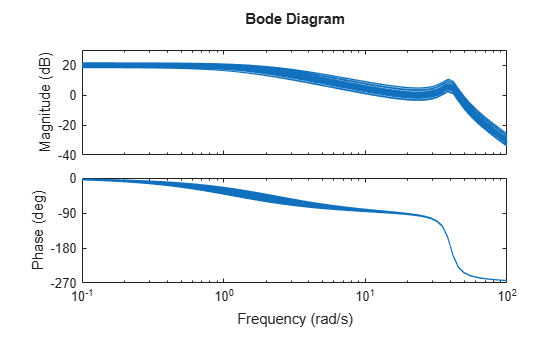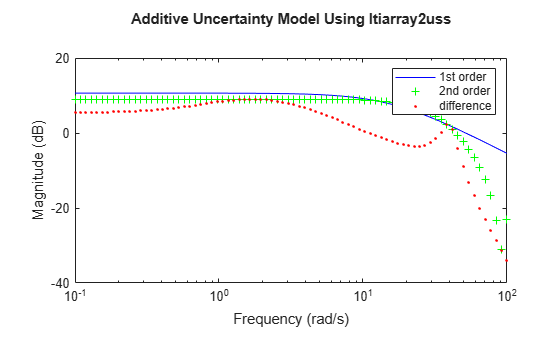Documentation

# ltiarray2uss

Compute uncertain system bounding given LTI `ss` array

## Compatibility

### Note

`ltiarray2uss` will be removed in a future release. Use `ucover` instead.

## Synopsis

`usys = ltiarray2uss(P,Parray,ord)`

```[usys,wt] = ltiarray2uss(P,Parray,ord)```

```[usys,wt,diffdata] = ltiarray2uss(P,Parray,ord)```

```[usys,wt,diffdata] = ltiarray2uss(P,Parray,ord,'InputMult')```

```[usys,wt,diffdata] = ltiarray2uss(P,Parray,ord,'OutputMult')```

```[usys,wt,diffdata] = ltiarray2uss(P,Parray,ord,'Additive')```

## Description

The command `ltiarray2uss`, calculates an uncertain system `usys` with nominal value `P`, and whose range of behavior includes the given array of systems, `Parray`.

`usys = ltiarray2uss(P,Parray,ord), usys` is formulated as an input multiplicative uncertainty model,

```usys = P*(I + wt*ultidyn('IMult',[size(P,2) size(P,2)]))```, where `wt` is a stable scalar system, whose magnitude overbounds the relative difference, `(P - Parray)/P`. The state order of the weighting function used to bound the multiplicative difference between `P` and `Parray` is `ord`. Both `P` and `Parray` must be in the classes `ss/tf/zpk/frd`. If `P` is an `frd` then `usys` will be a `ufrd` object, otherwise `usys` will be a `uss` object. The `ultidyn` atom is named based on the variable name of `Parray` in the calling workspace.

`[usys,wt] = ltiarray2uss(P,Parray,ord)`, returns the weight `wt` used to bound the infinity norm of ```((P - Parray)/P)```.

```[usys,wt] = ltiarray2uss(P,Parray,ord,'OutputMult')```, uses multiplicative uncertainty at the plant output (as opposed to input multiplicative uncertainty). The formula for `usys` is

```usys = (I + wt*ultidyn('Name',[size(P,1) size(P,1)])*P)```.

```[usys,wt] = ltiarray2uss(P,Parray,ord,'Additive')```, uses additive uncertainty.

```usys = P + wt*ultidyn('Name',[size(P,1) size(P,2)])```. `wt` is a frequency domain overbound of the infinity norm of `(Parray - P)`.

```[usys,wt] = ltiarray2uss(P,Parray,ord,'InputMult')```, uses multiplicative uncertainty at the plant input (this is the default). The formula for `usys` is ```usys = P*(I + wt*ultidyn('Name',[size(P,2) size(P,2)]))``` .

```[usys,wt,diffdata] = ltiarray2uss(P,Parray,ord,type)``` returns the norm of the difference (absolute difference for additive, and relative difference for multiplicative uncertainty) between the nominal model `P` and `Parray`. `wt` satisfies ```diffdata(w_i) < |wt(w_i)|``` at all frequency points.

## Examples

collapse all

Consider a third order transfer function with an uncertain gain, filter time constant and a lightly damped flexible mode. This model is used to represent a physical system from which frequency response data is acquired.

```gain = ureal('gain',10,'Perc',20); tau = ureal('tau',.6,'Range',[.42 .9]); wn = 40; zeta = 0.1; usys = tf(gain,[tau 1])*tf(wn^2,[1 2*zeta*wn wn^2]); sysnom = usys.NominalValue; parray = usample(usys,30); om = logspace(-1,2,80); parrayg = frd(parray,om); bode(parrayg)```The frequency response data in `parray` represents 30 experiments performed on the system. The command `ltiarray2uss` is used to generate an uncertain model, `umod`, based on the frequency response data. Initially an input multiplicative uncertain model is used to characterize the collection of 30 frequency responses. First and second order input multiplicative uncertainty weight are calculated from the data.

```[umodIn1,wtIn1,diffdataIn] = ltiarray2uss(sysnom,parrayg,1); [umodIn2,wtIn2,diffdataIn] = ltiarray2uss(sysnom,parrayg,2); bodemag(wtIn1,'b-',wtIn2,'g+',diffdataIn,'r.',om) title('Input Multiplicative Uncertainty Model Using ltiarray2uss') legend('1st order','2nd order','difference','Location','SouthEast')```Alternatively, an additive uncertain model is used to characterize the collection of 30 frequency responses.

```[umodAdd1,wtAdd1,diffdataAdd] = ltiarray2uss(sysnom,parrayg,1,'Additive'); [umodAdd2,wtAdd2,diffdataAdd] = ltiarray2uss(sysnom,parrayg,2,'Additive'); bodemag(wtAdd1,'b-',wtAdd2,'g+',diffdataAdd,'r.',om) title('Additive Uncertainty Model Using ltiarray2uss') legend('1st order','2nd order','difference')```